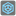• # In my Python EDL it works

## Question related to missionCount Inversions

I would like to give some feedback about ...

HTTP_USER_AGENT:

```Mozilla/5.0 (Windows NT 6.1) AppleWebKit/537.36 (KHTML, like Gecko) Chrome/37.0.2062.120 Safari/537.36
```

My Code:

```def count_inversion(seq, k=0):
seq=list(seq)
for x in range(len(seq)-1):
if seq[x]>seq[x+1]:
seq[x],seq[x+1] = seq[x+1], seq[x]
return count_inversion(seq, k+1)
return k

if __name__ == '__main__':
#These "asserts" using only for self-checking and not necessary for auto-testing
assert count_inversion((1, 2, 5, 3, 4, 7, 6)) == 3, "Example"
assert count_inversion((0, 1, 2, 3)) == 0, "Sorted"
assert count_inversion((99, -99)) == 1, "Two numbers"
assert count_inversion((5, 3, 2, 1, 0)) == 10, "Reversed"
```15